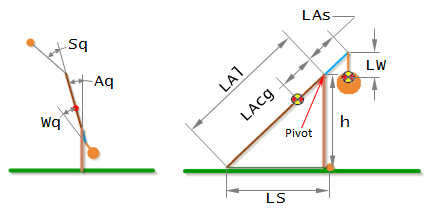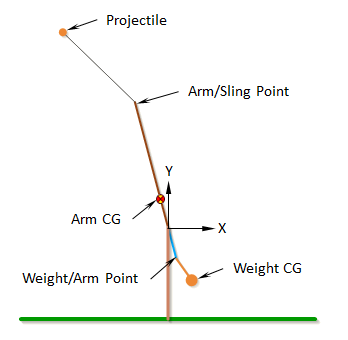# Angles to XY Coordinates

The equations of motion for the first two stages of the trebuchet given in the Equations of Motion section are used to find the angles (Aq, Wq, Sq) of the trebuchet over time. These angles can be used to find the Cartesian coordinates of the different parts of the trebuchet.Weight CG:

`X = LAs*SIN(Aq) + LW*SIN(Aq+Wq)`

`Y = -LAs*COS(Aq) - LW*COS(Aq+Wq)`

Weight/Arm Point:

`X = LAs*SIN(Aq)`

`Y = -LAs*COS(Aq)`

Arm CG:

`X = -LAcg*SIN(Aq)`

`Y = LAcg*COS(Aq)`

Arm/Sling Point:

`X = -LAl*SIN(Aq)`

`Y = LAl*COS(Aq)`

Projectile:

`X = -LAl*SIN(Aq) - LS*SIN(Aq+Sq)`

`Y = LAl*COS(Aq) + LS*COS(Aq+Sq)`

 Units English (feet)English (inches)Metric Length of Short Arm ft Length of Long Arm ft Length of Sling ft Length of Weight ft Height of Pivot ft Uniform arm Mass of Arm lb Inertia of Arm lb·ft² Pivot to Arm CG ft Mass of Weight lb Inertia of Weight lb·ft² Projectile BaseballGolf BallPumpkinCowCustom Mass of Projectile lb Projectile Diameter ft Wind Speed ft/s Release Angle ° Play speed: 1x Link to save this trebuchet

## VirtualTrebuchet 2.0

VirtualTrebuchet is a web based trebuchet simulator that will allow you to quickly evaluate different trebuchet configurations.

1. To begin, enter the parameters of your trebuchet in the input boxes.
2. Next, press the "Simulate" button located under the inputs.
3. Finally, watch your trebuchet go.